# Count the number of cells with numeric data in a range in Microsoft Excel

In this chapter, you’ll learn about how to count the number of cells with numeric data in a range in Microsoft Excel. We will use COUNT function.

COUNT function is used for counting the number of cells within a row, column and defined range.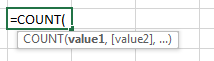Count the number within column:

Let’s take an example and understand:-

We have data in a column in which we have few blank cells, few cells contain text and few cells are have numbers. So, in this column we want to calculate number cells.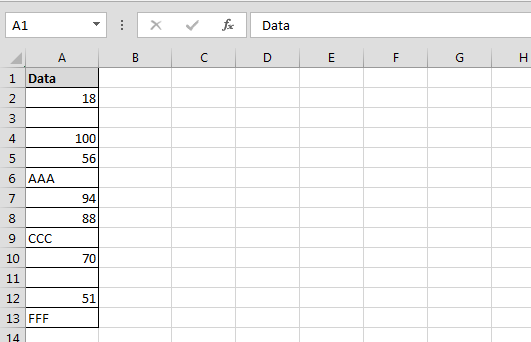• Enter the formula in cell B2
• =COUNT(A2:A13)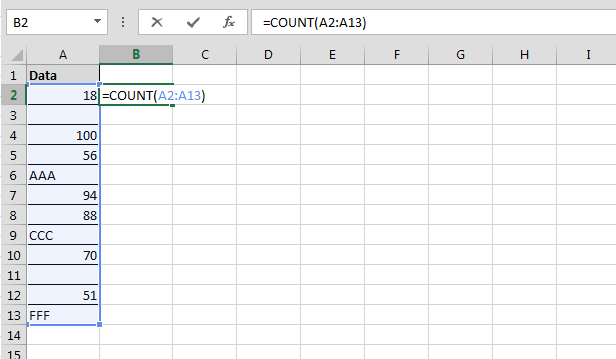• Press Enter
• The function will return 7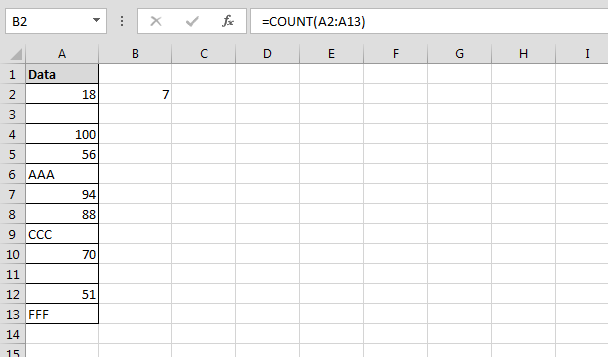Count the number within Row:

Let’s take an example and understand:-

We have data in row in which we have few blank cells, few cells are containing text and few cells are having numbers. So, in this row we want to calculate numbers cells.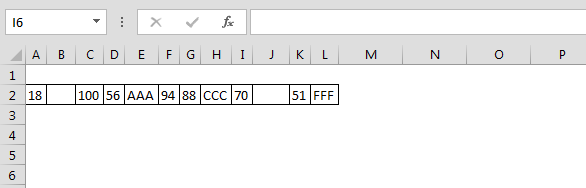• Enter the formula in cell A3
• =COUNT(A2:L2)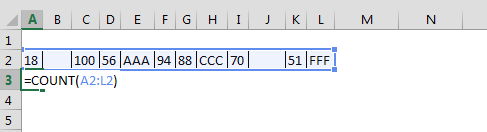• Press Enter
• The function will return 7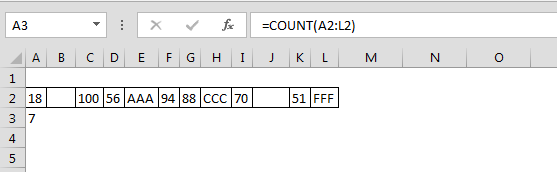Count the number within Row:

Let’s take an example and understand:-

We have data in the range A1:C10, same problem we have in the data. Few cells contain the numbers, few contain text and few cells are blank.

How we count the number within a range:-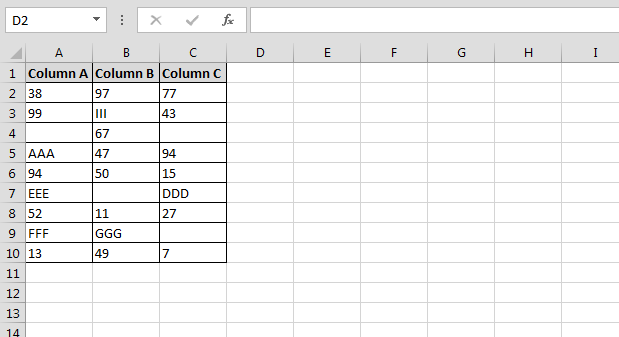• Enter the formula in cell D2
• =COUNT(A2:C10)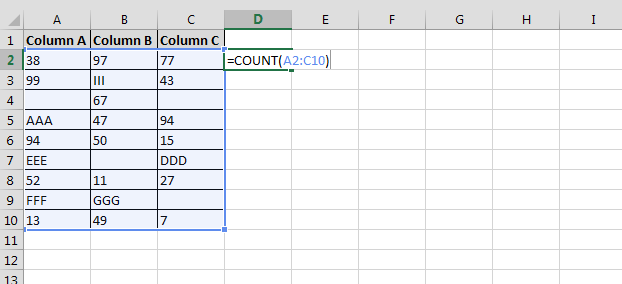• Press Enter
• The function will return 17, it means 17 cells are containing the numbers within range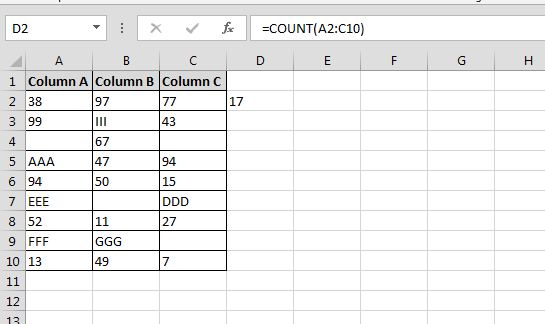We would love to hear from you, do let us know how we can improve, complement or innovate our work and make it better for you. Write us at info@exceltip.com

1.Im using COUNT successfully but ran into a problem when trying to use dynamic named range with it. For some reason if I just use count it calculates perfectly. If I setup a dynamic named ranged and apply it in the formula it reduces the number of the count. Im Using OFFSET(SHEETNAME!\$STARTCELL,0,0,COUNTA(range),1)

Any ideas what Im doing wrong or what I should be doing differently perhaps?

Thanks,
D

•if you are naming data in column A as "data" starting from A5 using below formula in named range.
=OFFSET(Sheet1!\$A\$5,0,0,COUNTA(Sheet1!\$A:\$A),1)
then
=count(data) will give you the correct answer.

if it doesn't help, kindly discribe the problem.

Terms and Conditions of use

The applications/code on this site are distributed as is and without warranties or liability. In no event shall the owner of the copyrights, or the authors of the applications/code be liable for any loss of profit, any problems or any damage resulting from the use or evaluation of the applications/code.